﻿ 20-sim webhelp > Library > Signal > Transfer Functions > TransferFunctionWithDeadTime

## Library

Signal\Transfer Functions

ZOD

FOD

SOID

SOOD

SOUD

SOOLD

SOUDO

## Use

Domains: Discrete, Continuous. Size: 1-D. Allowed in: Block Diagrams.

## Description - ZOD

This model describes a first zero process plus dead time determined by k and theta. The transfer function of this process is: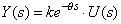Inputs

Description

input

output

## Parameters

k

theta

Proportional gain [].

## Description - FOD

This model describes a first order process plus dead time determined by k tau and theta. The transfer function of this process is: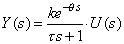Inputs

Description

input

output

## Initial Values

state_intitial

The initial value of the output after the dead time has passed.

## Parameters

k

tau

theta

Proportional gain [].

Process time constant [s].

## Description - SOID

This model describes a second order process with integrator plus dead time determined by the parameters k and tau. The transfer function of this process is: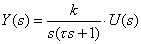Inputs

Description

input

output

## Initial Values

state1_initial

state2_initial

Internal state.

The initial value of the output after the dead time has passed.

## Parameters

k

tau

theta

Proportional gain [].

Process time constant [s].

## Description - SOOD

This model describes an overdamped second order process plus dead time determined by the parameters k, tau1 and tau2. The transfer function of this process is: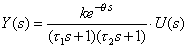Inputs

Description

input

output

## Initial Values

state1_initial

state2_initial

Internal state.

The initial value of the output after the dead time has passed.

## Parameters

k

tau1

tau2

theta

Proportional gain [].

First process time constant [s].

Second process time constant [s].

## Description - SOUD

This model describes an underdamped second order process plus Dead Time, determined by the parameters k, tau and zeta. The transfer function of this process is: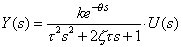Inputs

Description

input

output

## Initial Values

state1_initial

state2_initial

Internal state.

The initial value of the output after the dead time has passed.

## Parameters

k

tau

zeta

theta

Proportional gain [].

Natural period of oscillation [s].

Damping factor []

## Description - SOOLD

This model describes an overdamped second order process with lead time plus dead time determined by the parameters k, tau1, tau2 and tauL. The transfer function of this process is: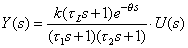Inputs

Description

input

output

## Initial Values

state1_initial

state2_initial

Internal state.

The initial value of the output after the dead time has passed.

## Parameters

k

tau1

tau2

tauL

theta

Proportional gain [].

First process time constant [s].

Second process time constant [s].

## Description - SOUDO

This model describes an underdamped second order process plus Dead Time, determined by the parameters k, omega and zeta. The transfer function of this process is: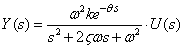Inputs

Description

input

output

## Initial Values

state1_initial

state2_initial

Internal state.

The initial value of the output after the dead time has passed.

## Parameters

k

omega

zeta

theta

Proportional gain [].